}

# How to Interpret a Q-Q Plot

6/4/2021

Statisticians have developed a remarkably powerful set of tools for analyzing normally distributed data. Too bad real data is never normally distributed.

Fortunately for us, most of the time "close enough" is all we really need. But how are we to know? One quick and effective method is a look at a Q-Q plot. The Q's stand for "quantile" and a Q-Q plot. Technically speaking, a Q-Q plot compares the distribution of two sets of data. In most cases, a probability plot will be most useful. A probability plot compares the distribution of a data set with a theoretical distribution. The R function qqnorm( ) compares a data set with the theoretical normal distibution.

We can start by looking at the mpg column of the familiar mtcars sample dataframe.

`qqnorm(mtcars\$mpg)qqline(mtcars\$mpg, col = "steelblue", lwd = 2)`

The qqline( ) function plots a line representing perfect quantile matching.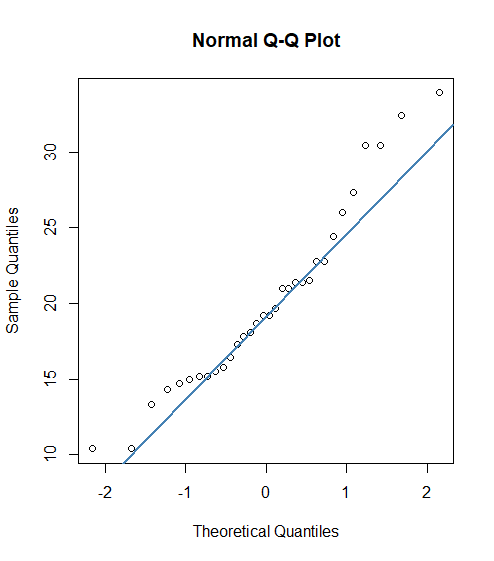If the distributions matched perfectly, all the quantile points would lie along the blue line. Is the deviation we see here cause for concern?

Let's generate some normally distributed random numbers and see how they look on a probability plot.

dfN1<-rnorm(1000, mean=50, sd = 10)

qqnorm(dfN1)

qqline(dfN1, col="maroon4", lwd=2) # there is no maroon five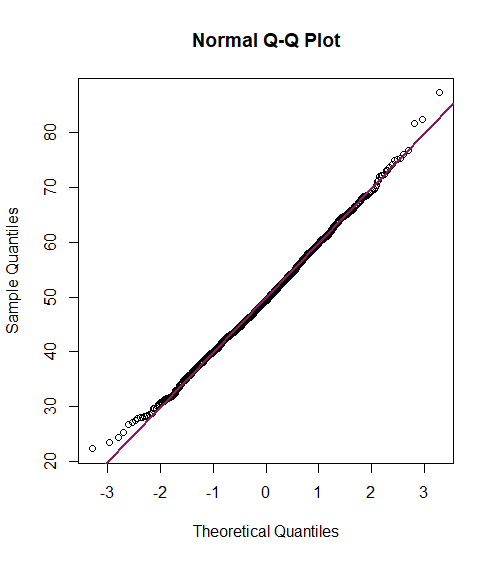Since a relatively small number of data points in normally distributed data fall in the few highest and few lowest quantiles, we are more likely to see the results of random fluctuations at the extreme ends. We now understand that the mtcars mpg data is not precisely normal, but not too far off.

Now let's generate some sample random data that we know not to be normal. We can do this using the sn package.

library(sn)

y3 <- dsn(x, xi=0, omega=1.2, alpha=2)

plot(x, y3, type="l", ylab="density", col="royalblue")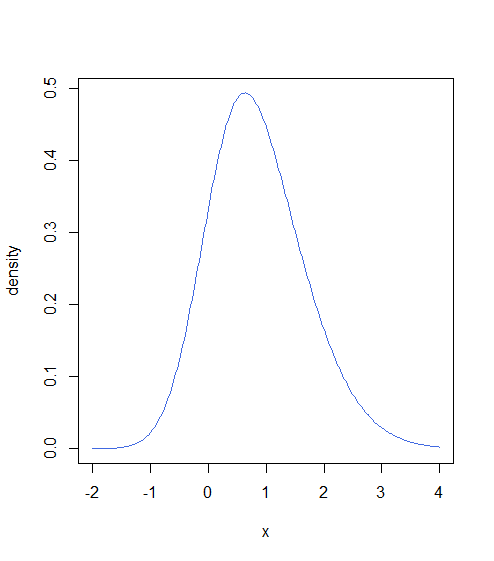This dataset is not normally distributed, but doesn't look that far off. Let's take a look at the output of qqnorm( ) for this data.

qqnorm(y3)

qqline(y3, col = "dodgerblue4", lwd = 2)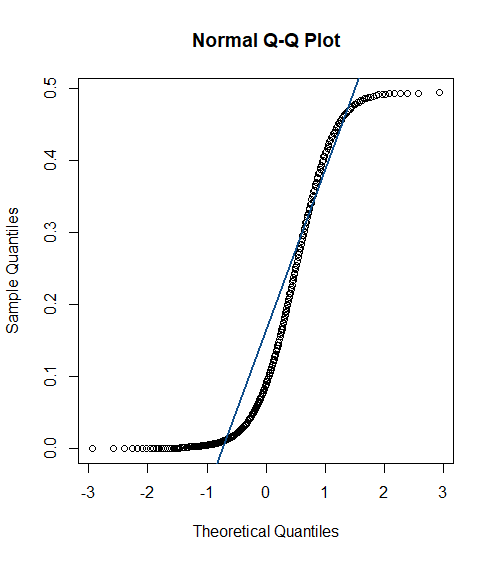The Q-Q plot clearly shows that the quantile points do not lie on the theoretical normal line. We see that the sample values are generally lower than the normal values for quantiles along the smaller side of the distribution.

### A True Q-Q Plot

It is very common to ask if a particular dataset is close to normally distributed, the task for which qqnorm( ) was designed. However, you may wish to compare the distribution of two datasets to see if the distributions are similar without making any further assumptions. R implements the qqplot( ) for this purpose. Unfortunately, since we are not comparing to any theoretical distribution in this case, there is nothing comparable to qqline( ) available in qqplot. We can, however, use abline( ) to draw the same line if we calculate the appropriate intercept and slope.
abline(intercept,slope)
Just out of curiosity we might compare samples following t-distributions with different values for degrees of freedom.

t20<-rt(1000,df=20)

t3<-rt(1000,df=3)

qqplot(t3,t20)

abline(0,sd(t20)/sd(t3), col="firebrick2")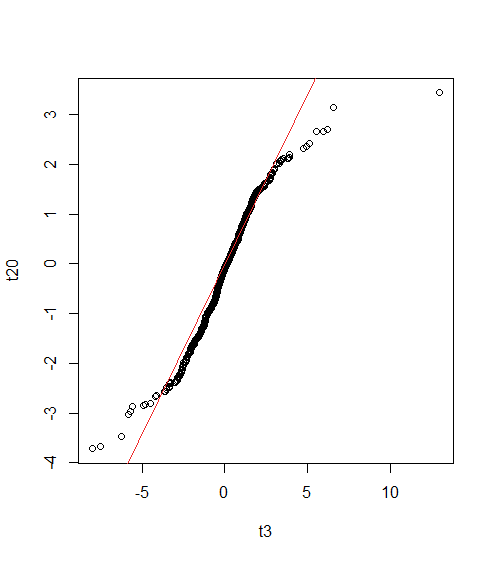Conclusion

As is so often the case in data science, well-chosen graphs communicate information more quickly and more understandably. Q-Q plots and probability plots provide quick comparisons between probability distributions and can tell us how closely a data sample is to normally distributed.### Written by Dan Buskirk

The pleasures of the table belong to all ages.” Actually, Brillat-Savaron was talking about the dinner table, but the quote applies equally well to Dan’s other big interest, tables of data. Dan has worked with Microsoft Excel since the Dark Ages and has utilized SQL Server since Windows NT first became available to developers as a beta (it was 32 bits! wow!). Since then, Dan has helped corporations and government agencies gather, store, and analyze data and has also taught and mentored their teams using the Microsoft Business Intelligence Stack to impose order on chaos. Dan has taught Learning Tree in Learning Tree’s SQL Server & Microsoft Office curriculums for over 14 years. In addition to his professional data and analysis work, Dan is a proponent of functional programming techniques in general, especially Microsoft’s new .NET functional language F#. Dan enjoys speaking at .NET and F# user’s groups on these topics.

Chat With Us